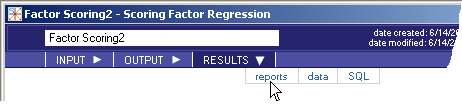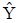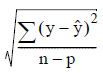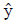# 5.4.5 - Factor Scoring - RESULTS - Reports - Teradata Warehouse Miner

## Teradata Warehouse Miner User Guide - Volume 3Analytic Functions

Product
Release Number
5.4.5
Published
February 2018
Language
English (United States)
Last Update
2018-05-04
dita:mapPath
yuy1504291362546.ditamap
dita:ditavalPath
ft:empty
1. On the Factor Scoring dialog box, click RESULTS.
2. Click reports (note that the RESULTS tab is disabled until after the analysis is completed).
Factor Scoring > Results > Reports• Resulting Scored Table — Name of the scored table - equivalent to Result Table Name.
• Number of Rows in Scored Table — Number of rows in the Resulting Scored Table.
• Evaluation — Model evaluation for factor analysis consists of computing the standard error of estimate for each variable based on working backwards and re-estimating their values using the scored factors. Estimated values of the original data are made using the factor scoring equation= XCT whereis the estimated raw data, X is the scored data, and C is the factor pattern matrix or rotated factor pattern matrix if rotation was included in the model. The standard error of estimate for each variable y in the original data Y is then given by:where eachis the estimated value of each variable y, n is the number of observations and p is the number of factors.